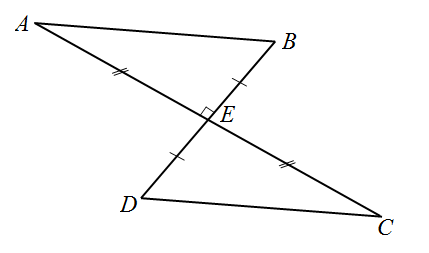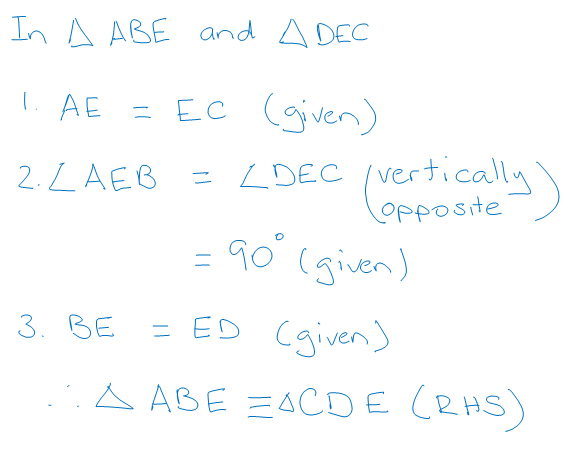Find the error

Thinking through a problem which involves geometric reasoning and then writing a formal proof can be a challenging task for students. If the proof contains errors it can be difficult to determine the misunderstanding or difficulty the student is actually experiencing.

To isolate misunderstandings more precisely, provide students with a proof which has an error. Ask them to find the error and correct it.

For example:

A student was asked to write the following proof:

In the diagram below, AE = EC and BE = DE. $$\angle$$AEB = 90°.

Prove that ΔABE $$\cong$$ ΔCDE.There are errors in the proof. What is wrong with the proof?